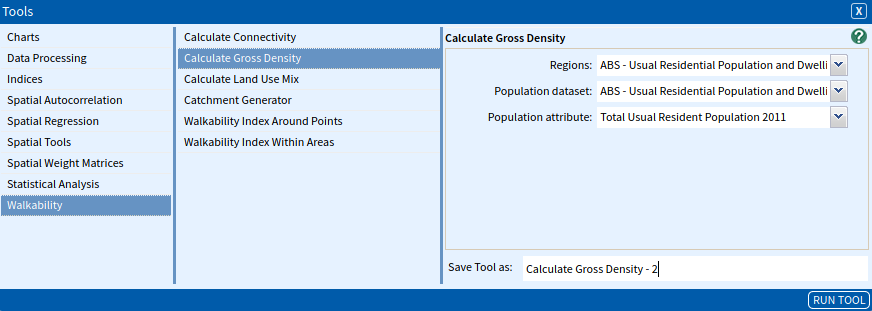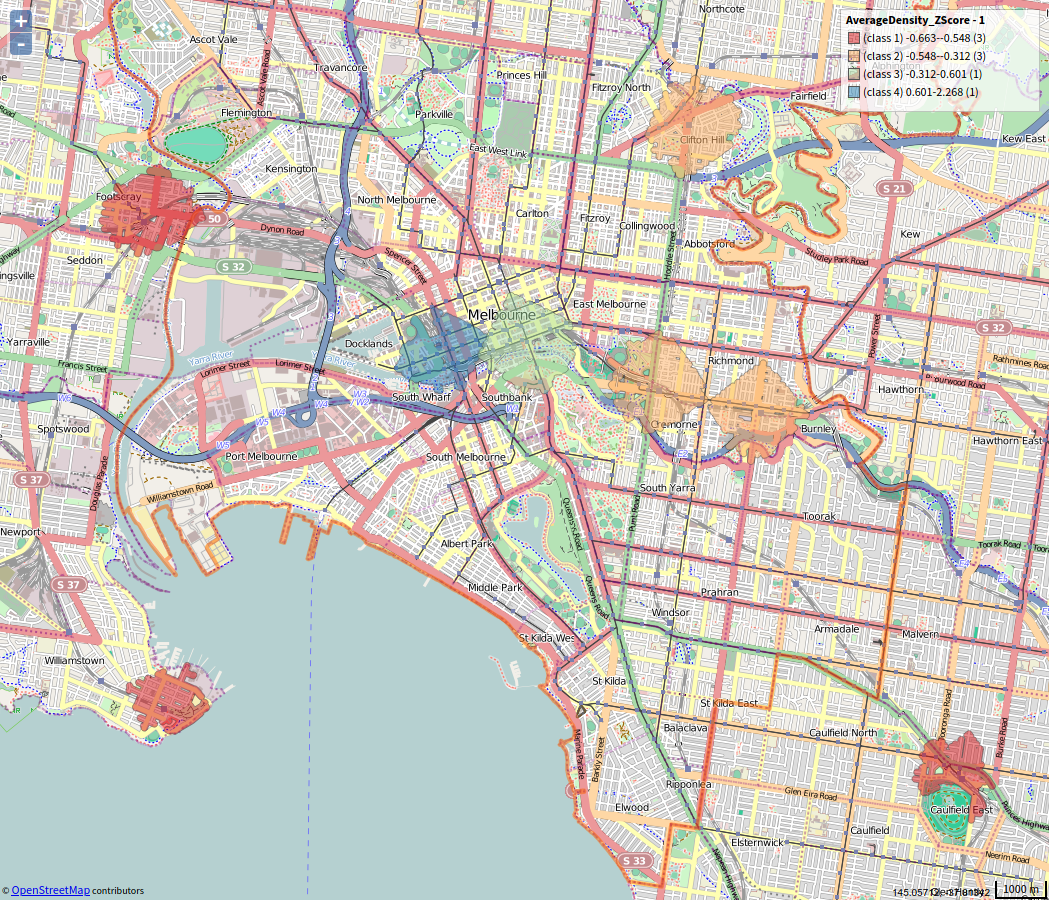Calculate Gross Density

The Calculate Gross Density tool allows you to calculate the average population density within walkability catchments across your study area. Alternatively, the tool can be used to calculate the population density within a polygon dataset of your choice. We will run both of the analyses here to show their differences.

Note: The walkability tools are designed to work on regions around the size of local government areas. Study areas larger than this may be faced with long processing times.

SET UP

Set up your area and data, for this example, we will look at Inner Melbourne SA4.

• Select Melbourne – Inner SA4 (Australia → Victoria → Greater Melbourne → Melbourne – Inner) as your area.
• Select OpenStreetMap – Lines (Australia) 2017 and select the following variables:
• Unique Identifier
• Geometry
• Select VIC DELWP – Vicmap Lite – Statewide Railway Stations (Points) and select the following variables:
• Object ID
• Geometry
• Select ABS – Usual Residential Population and Dwelling Count (MB) 2011 and select the following variables:
• Mesh Block Code
• Total Usual Resident Population 2011
• Geometry

Note: The walkability tools work optimally on regions around the size of local government areas. Study areas larger than this may be faced with long processing times.

Inputs

1. Walkability catchment density

We will first look at calculating the average density of walking catchments around statewide railway stations within Melbourne. You will first need to use the Catchment Generator tool to generate neighbourhoods around the railway stations selecting 800m as your walking distance and 50m as your trim distance.

Once you have done this, open the Calculate Gross Density tool (Tools → Walkability → Calculate Gross Density) and enter the parameters as follows:

• Regions: The catchment areas that you would like to include for your analysis. Select the output created from the Catchment Generator tool.
• Population dataset: This is the dataset that contains your population count. Select ABS – Usual Residential Population and Dwelling Count (MB) 2011.
• Population attribute: This is the column that contains your population count. Select Total Usual Resident Population 2011.

Once you have entered your parameters, click Run Tool.2. Mesh Block Density

To calculate the mesh-block density, we can use the tool just as we have above. However, when choosing Regions we choose the same as our population dataset. Select ABS – Usual Residential Population and Dwelling Count (MB) 2011.Again, once you have entered your parameters, click Run Tool.

Outputs

1. Walkability catchment density

The outputs of the tool provide you with the following table.The final two columns represent the Average Density in people per hectare for each of the walkability catchments, and the Z score for that value, where the mean for the different catchments is zero, and the numbers indicate how many standard deviations each score is above or below the mean. Essentially, the more positive the number, the better the relative score for that attribute, and the more negative number, the worse the relative score for that attribute. This is represented in the image below and is calculated by the formula;

$Z_{i} = {X_{i} - \overline{X}}\over{s}$

Where $X_{i}$ is the individual score for observation $i$, $\overline{X}$ is the mean of all the scores, and $s$ represents the sample standard deviation.Note: We recommend that you make sure you have a relatively large number of observations (a minimum of 30) before using Z scores in any discussion, as they rely on robust mean and standard deviation calculations, which are less reliable at smaller samples sizes.

If you create a visualisation with the Choropleth tool of the Z scores (using a Diverging palette) it should look something like the image below, where we can see that the average density tends to be higher towards the state railway stations in the CBD of Melbourne of the study area and lower into the suburbs.2. Mesh Block Density

The outputs of this routine are the same as above, however, the density calculation has been processed for every meshblock producing an area of each meshblock and thus generating a density of people per hectare.

We are interested in looking at the spatial distribution of the density. The image below represents the Average Density Z-Score for each mesh block in Melbourne.Looking for Spatial Data?

You can browse the AURIN Data Discovery:How can you Create Impact?